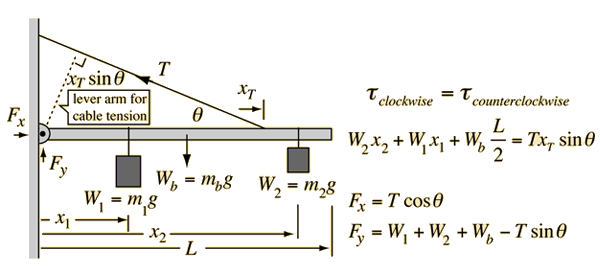# Support of a Boom

The support of a pivoted, uniform boom with a cable is a standard exercise in equilibrium of torques. Using a pivot at the wall, which is assumed to exert no torque, the torque equation is that shown below. The forces exerted on the boom are then obtainable from the force equation shown. The lever arm for the cable tension T must be obtained from the triangle as shown since the cable is not perpendicular to the boom.A uniform boom of length L=m, mass kg and weight N
is used to support a load of mass m1 = kg and weight W1 = N
at a distance of x1=m
and a second load of mass m2 = kg and weight W2 = N
at a distance of x2=m.
The boom is supported by a cable which is attached at x=m and acting at an angle of degrees with respect to the boom.

### The required tension in the cable is N.

In this loaded condition the pivot at the wall must exert a horizontal force of N outward from the wall and a vertical force of N.
The required tension is recalculated when any change is made in the above data. Check the change in required cable tension when you make changes. Does the change in tension make sense to you?
Index

Torque equilibrium examples

 HyperPhysics***** Mechanics R Nave
Go Back